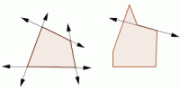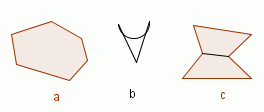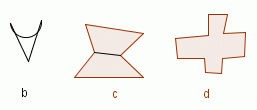Email us to get an instant 20% discount on highly effective K-12 Math & English kwizNET Programs!

#### Online Quiz (WorksheetABCD)

Questions Per Quiz = 2 4 6 8 10

### Geometry2.1 Triangles and Polygons

 Polygons: A simple closed figure bound by line segments is called a polygon. They are two dimensional figures. The line segments by which a polygon is bound are called its sides. The sides do not cross each other and exactly two sides meet at every vertex.A polygon is convex if no line that contains a side of the polygon contains a point in the interior of the polygon. A polygon that is not convex is called nonconvex or concave.In an equilateral polygon, all sides are congruent. In an equiangular polygon, all angles in the interior of the polygon are congruent. A regular polygon is a convex polygon that is both equilateral and equiangular. Polygons with three sides are called triangles. A triangle is named by its vertices. If D, E, and F are the vertices of a triangle, it is called triangle DEF. The symbol for a triangle isand is read as "Triangle". "DEF" is read as "Triangle DEF". Polygons with four sides are called quadrilaterals. A quadrilateral with two pairs of parallel and equal sides is called a parallelogram. A parallelogram with 4 right angles and 4 sides equal is a square. A parallelogram with 4 right angles and 2 pairs of equal sides is a rectangle. A quadrilateral with only 1 pair of parallel sides is called trapezoid. The diagonals of a parallelogram are not equal. A polygon that has 5 sides is a pentagon. A polygon that has 6 sides is a hexagon. A polygon that has 7 sides is a heptagon. A polygon that has 8 sides is a octagon. A polygon that has 9 sides is a nonagon. A polygon that has 10 sides is a decagon. A polygon that has 12 sides is a dodecagon. A polygon that has n sides is a n-gon. A solid formed by plane figures is a polyhedron. Cube is an example of a polyhedron. Directions: On a sheet of paper draw the following: * At least 10 examples of polygons and name their properties. * 5 convex polygons and 5 concave polygons * 5 regular polygons * 5 figures which are not polygons and explain why. * A convex triangle, a regular polygon that is equiangular, triangle that is not regular, pentagon that is equilateral but not equiangular Select if the following statements are true or false.
 Q 1: A circle is a polygon.FalseTrue Q 2: All triangles are polygons.TrueFalse Q 3: Which figure is convex polygon?bca Q 4: Which figure is concave polygon?dbc Q 5: Which figure is convex polygon?ghef Q 6: If A, B, and C are three points, then the union of AB, BC and CA is triangle ABC.TrueFalse Question 7: This question is available to subscribers only! Question 8: This question is available to subscribers only!